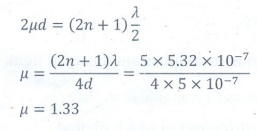Home | | Physics 12th Std | Multiple choice questions

# Multiple choice questions

Wave Optics | Physics : Book Back Choose the correct Answers with Solution, Multiple choice questions with Answers and Solution

Multiple choice questions

1. A plane glass is placed over a various coloured letters (violet, green, yellow, red) The letter which appears to be raised more is,

(a) red

(b) yellow

(c) green

(d) violet

Solution:

The refractive index (n) of the glass is different for different colours. For violet n is high.

Violet travel with low speed, so deviation is high.

2. Two point white dots are 1 mm apart on a black paper. They are viewed by eye of pupil diameter 3 mm approximately. The maximum distance at which these dots can be resolved by the eye is, [take wavelength of light, λ = 500 nm]

(a) 1 m

(b) 5 m

(c) 3 m

(d) 6m

Solution:

Resolution limit = 1.22 x λ / D

D - diameter of pupil = 3 mm

λ - wavelength = 500 nm

Resolution limit = distance between the dots / resolving diameter

= 1mm/d

[ 1.22 × λ ] / D =  1mm / d

D = [1mm × D ] / [ 1.22 × λ ] = [ 1 x 10-3 x 3 x 10-3 ] / [ 1.22 x500 x10-9 ]

d = 4.918 ≈ 5m

3. In a Young’s double-slit experiment, the slit separation is doubled. To maintain the same fringe spacing on the screen, the screen-to-slit distance D must be changed to,

(a) 2D

(b) D/2

(c) √2D

(d) D/√2

Solution:4. Two coherent monochromatic light beams of intensities I and 4I are superposed. The maximum and minimum possible intensities in the resulting beam are

(a) 5I and I

(b) 5I and 3I

(c) 9I and I

(d) 9I and 3I

Solution:

Imax = I1 + I2 + 2√(I1I2)

= I + 4I + 2√(4I2)

= I + 4I + 4I = 9I

Imin = I1 + I2 - 2√(I1I2)

= I + 4I − 2√(4I2)

= I + 4I − 4I = I

5. When light is incident on a soap film of thickness 5×10–5 cm, the wavelength of light reflected maximum in the visible region is 5320 Å. Refractive index of the film will be,

(a) 1.22

(b) 1.33

(c) 1.51

(d) 1.83.

Solution:

d = 5 × 10-5 cm = 5 × 10-7 m

λ=5320 Å = 5.32 × 10-7 m

For visible region n=2

μ=?6. First diffraction minimum due to a single slit of width 1.0×10–5 cm is at 30º. Then wavelength of light used is,

(a) 400 Å

(b) 500 Å

(c) 600 Å

(d) 700 Å

Solution:

λ = a sin θ = 1 x 10-7 x sin 30° =500 Å

7. A ray of light strikes a glass plate at an angle 60º. If the reflected and refracted rays are perpendicular to each other, the refractive index of the glass is,

(a) √3

(b) 3/2

(c) √(3/2)

(d) 2

Solution: n = tan ip = tan (600) = √3

8. One of the of Young’s double slits is covered with a glass plate as shown in figure. The position of central maximum will,(a) get shifted downwards

(b) get shifted upwards

(c) will remain the same

(d) data insufficient to conclude

9. Light transmitted by Nicol prism is,

(a) partially polarised

(b) unpolarised

(c) plane polarised

(d) elliptically polarised

10. The transverse nature of light is shown in,

(a) interference

(b) diffraction

(c) scattering

(d) polarisation